# Modern SAT solvers: fast, neat and underused (part 1 of N)

Modern SAT solvers: fast, neat and underused (part 1 of N)

By Martin Hořeňovský

SAT solvers can quickly find solutions to Boolean Logic problems. Martin Hořeňovský demonstrates how this can be used to solve arbitrary Sudoku puzzles.

Before I started doing research for Intelligent Data Analysis (IDA) group at FEE CTU, I saw SAT solvers as academically interesting but didn’t think that they have many practical uses outside of other academic applications. After spending ~1.5 years working with them, I have to say that modern SAT solvers are fast, neat and criminally underused by the industry.

## Introduction

Boolean satisfiability problem (SAT) is the problem of deciding whether a formula in boolean logic is satisfiable. A formula is satisfiable when at least one interpretation (an assignment of ``` true ``` and ``` false ``` values to logical variables) leads to the formula evaluating to ``` true ``` . If no such interpretation exists, the formula is unsatisfiable .

What makes SAT interesting is that a variant of it was the first problem to be proven NP-complete, which roughly means that a lot of other problems can be translated into SAT in reasonable 1 time, and the solution to this translated problem can be converted back into a solution for the original problem.

As an example, the often-talked-about dependency management problem is also NP-Complete and thus translates into SAT 2 , 3 , and SAT could be translated into dependency manager. The problem our group worked on, generating key and lock cuttings based on user-provided lock-chart and manufacturer-specified geometry, is also NP-complete.

To keep this article reasonably short, we will leave master-key systems for another article and instead use Sudoku for practical examples.

## Using SAT solvers

These days, SAT almost always refers to CNF-SAT 4 , a boolean satisfaction problem for formulas in conjunctive normal form (CNF) [ Wikipedia-1 ]. This means that the entire formula is a conjunction (AND) of clauses, with each clause being a disjunction (OR) of literals. Some examples:

• (A ˅ B) ˄ (B ˅ C)
• (A ˅ B) ˄ C
• A ˅ B
• A ˄ C

There are two ways to pass a formula to a SAT solver: by using a semi-standard file format known as DIMACS, or by using the SAT solver as a library. In real-world applications, I prefer using SAT solver as a library (e.g. MiniSat for C++ [ MiniSAT ]), but the DIMACS format lets you prototype your application quickly, and quickly test the performance characteristics of different solvers on your problem.

## DIMACS format

DIMACS is a line oriented format, consisting of 3 different basic types of lines.

1. A comment line. Any line that starts with ``` c ``` is comment line.
2. A summary line. This line contains information about the kind and size of the problem within the file. A summary line starts with ``` p ``` , continues with the kind of the problem (in most cases, ``` cnf ``` ), the number of variables and the number of clauses within this problem. Some DIMACS parsers expect this line to be the first non-comment line, but some parsers can handle the file without it.
3. A clause line. A clause line consists of space-separated numbers, ending with a ``` 0 ``` . Each non-zero number denotes a literal, with negative numbers being negative literals of that variable, and ``` 0 ``` being the terminator of a line.

As an example, this formula

(A ˅ B ˅ C) ˄ (¬A ˅ B ˅ C) ˄ (A ˅ ¬B ˅ C) ˄ (A ˅ B ˅ ¬C)

would be converted into DIMACS as

```    c An example formula
c
p cnf 3 4
1 2 3 0
-1 2 3 0
1 -2 3 0
1 2 -3 0```

## Minisat’s C++ interface

MiniSat is a fairly simple and performant SAT solver that also provides a nice C++ interface and we maintain a modernised fork with CMake integration. The C++ interface to MiniSat uses 3 basic vocabulary types:

• ``` Minisat::Solver ``` – Implementation of the core solver and its algorithms.
• ``` Minisat::Var ``` – Representation of a variable.
• ``` Minisat::Lit ``` – Representation of a concrete (positive or negative) literal of a variable.

The difference between a variable and a literal is that the literal is a concrete ‘evaluation’ of a variable inside a clause. As an example, formula ( A ˅ B ˅ ¬ C ) ˄ (¬ A ˅ ¬ B ) contains 3 variables, A , B and C , but it contains 5 literals, A, ¬A, B, ¬B and ¬C.

MiniSat’s interface also uses one utility type: ``` Minisat::vec<T> ``` , a container similar to ``` std::vector ``` , that is used to pass clauses to the solver.

The example in Listing 1 uses MiniSat’s C++ API to solve the same clause as we used in the DIMACS example.

 ```// main.cpp: #include #include int main() { using Minisat::mkLit; using Minisat::lbool; Minisat::Solver solver; // Create variables auto A = solver.newVar(); auto B = solver.newVar(); auto C = solver.newVar(); // Create the clauses solver.addClause( mkLit(A), mkLit(B), mkLit(C)); solver.addClause(~mkLit(A), mkLit(B), mkLit(C)); solver.addClause( mkLit(A), ~mkLit(B), mkLit(C)); solver.addClause( mkLit(A), mkLit(B), ~mkLit(C)); // Check for solution and retrieve model if found auto sat = solver.solve(); if (sat) { std::clog << "SAT\n" << "Model found:\n"; std::clog << "A := " << (solver.modelValue(A) == l_True) << '\n'; std::clog << "B := " << (solver.modelValue(B) == l_True) << '\n'; std::clog << "C := " << (solver.modelValue(C) == l_True) << '\n'; } else { std::clog << "UNSAT\n"; return 1; } } ``` Listing 1

Because all of our clauses have length ≤ 3, we can get away with just using utility overloads that MiniSat provides, and don’t need to use ``` Minisat::vec ``` for the clauses.

We will also need to build the binary. Assuming you have installed our fork of MiniSat (either from GitHub [ MiniSAT ] or from vcpkg [ vcpkg ]), it provides proper CMake integration and writing the CMakeLists.txt is trivial (see Listing 2).

 ```cmake_minimum_required (VERSION 3.5) project (minisat-example LANGUAGES CXX) set(CMAKE_CXX_EXTENSIONS OFF) find_package(MiniSat 2.2 REQUIRED) add_executable(minisat-example main.cpp ) target_link_libraries(minisat-example MiniSat::libminisat) ``` Listing 2

Building the example and running it should 5 give you this output:

```  SAT
Model found:
A := 0
B := 1
C := 1```

## Conversion to CNF

Very few problems are naturally expressed as a logical formula in the CNF format, which means that after formulating a problem as a SAT, we often need to convert it into CNF. The most basic approach is to create an equivalent formula using De-Morgan laws, distributive law and the fact that two negations cancel out. This approach has two advantages: one, it is simple and obviously correct. Two, it does not introduce new variables. However, it has one significant disadvantage: some formulas lead to exponentially large CNF conversion.

The other approach is to create an equisatisfiable 6 CNF formula, but we won’t be covering that in this article.

Some common equivalencies are in Table 1.

Original clause Equivalent clause
¬¬ α α
α β ¬ α ˅ β
¬ ( α ˄ β ) ¬ α ˅ ¬ β
¬ (¬ α ˄ ¬ β ) α ˅ β
( α ˄ β ) ˅ γ ( α ˅ γ) ˄ ( β ˅ γ)
α β ( α β) ˄ β)
Table 1

Obviously, you don’t have to remember these identities, but knowing at least some of them (implication) is much faster than deriving them from the truth tables every time.

## Solving Sudoku using SAT

With this background, we can now look at how we could use a real-world problem, such as Sudoku, using a SAT solver. First, we will go over the rules of Sudoku [ Wikipedia-2 ] and how they can be translated into (CNF-)SAT. Then we will go over implementing this converter in C++ and benchmarking the results.

### Quick overview of Sudoku

Sudoku is a puzzle where you need to place numbers 1–9 into a 9 × 9 grid consisting of 9 3 × 3 boxes 7 , following these rules:

1. Each row contains all of the numbers 1–9
2. Each column contains all of the numbers 1–9
3. Each of the 3 × 3 boxes contains all of the numbers 1–9

We can also restate these rules as:

1. No row contains duplicate numbers
2. No column contains duplicate numbers
3. No 3 × 3 box contains duplicate numbers

Because these rules alone wouldn’t make for a good puzzle, some of the positions are pre-filled by the puzzle setter, and a proper Sudoku puzzle should have only one possible solution.

### Translating the rules

The first step in translating a problem to SAT is to decide what should be modelled via variables, and what should be modelled via clauses over these variables. With Sudoku, the natural thing to do is to model positions as variables, but in SAT, each variable can only have 2 values: ``` true ``` and ``` false ``` . This means we cannot just assign each position a variable, instead we have to assign each combination of position and value a variable. We will denote such variable as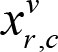. If variableis set to ``` true ``` , then the number in r -th row and c -th column is v .

Using this notation, let’s translate the Sudoku rules from the previous section into SAT.

#### Rule 1 (No row contains duplicate numbers)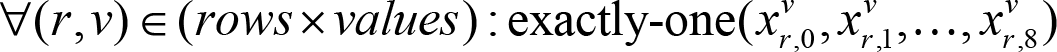In plain words, for each row and each value, we want exactly one column in that row to have that value. We do that by using a helper called exactly-one, that generates a set of clauses that ensure that exactly one of the passed-in literals evaluate to ``` true ``` .

We will see how to define exactly-one later. First, we will translate the other Sudoku rules into these pseudo-boolean formulas.

#### Rule 2 (No column contains duplicate numbers)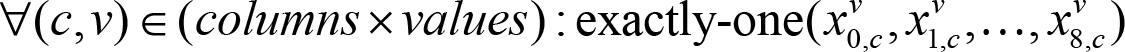This works analogically with Rule 1, in that for each column and each value, we want exactly one row to have that value.

#### Rule 3 (None of the 3x3 boxes contain duplicate numbers)

This rule works exactly the same way as the first two: for each box and each value, we want exactly one position in the box to have that value.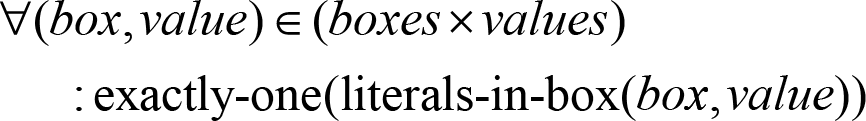Even though it seems to be enough at first glance, these 3 rules are in fact not enough to properly specify Sudoku. This is because a solution like this one:

0 1 2 3 4 5 6 7 8
0 x . . . . . . . .
1 . . . x . . . . .
2 . . . . . . x . .
3 . x . . . . . . .
4 . . . . x . . . .
5 . . . . . . . x .
6 . . x . . . . . .
7 . . . . . x . . .
8 . . . . . . . . x

where x denotes a position where all variables are set to ``` true ``` and . denotes a position where no variables are set to ``` true ``` , is valid according to the rules as given to the SAT solver.

This is because we operate with an unstated assumption, that each position can contain only one number. This makes perfect sense to a human, but the SAT solver does not understand the meaning of the variables, it only sees clauses it was given.

We can fix this simply by adding one more rule.

#### Rule 4 (Each position contains exactly one number)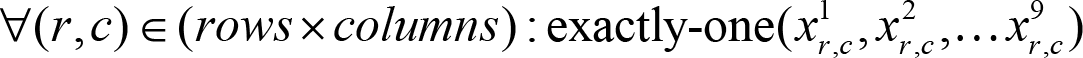With this rule in place, we have fully translated the rules of Sudoku into SAT and can use a SAT solver to help us solve sudoku instances. But before we do that, we need to define the helper our description of Sudoku relies on.

Unstated assumptions
 When translating problems into SAT, be very careful not to rely on unstated assumptions. While an assumption might seem common sense to a human, SAT solvers (and computers in general) do not operate on common sense, and will happily find a solution that is valid according to your specification of the problem but does not make sense in the context of human expectations.

## Exactly-one helper

There is no way to encode numeric constraints natively in boolean logic, but often you can decompose these constraints into simpler terms and encode these. Many research papers have been written about the efficient encoding of specific constraints and other gadgets, but in this article, we only need to deal with the most common and one of the simplest constraints possible: exactly one of this set of literals has to evaluate to true. Everyone who works with SAT often can write this constraint from memory, but we will derive it from first principles because it shows how more complex constraints can be constructed.

The first step is to decompose the constraint x == n into two parts: x n and x n , or for our specific case, x ≥ 1 and x ≤ 1, or, translated into the world of SAT, at least 1 literal has to evaluate to ``` true ``` , and no more than 1 literal can evaluate to ``` true ``` . Forcing at least one literal to be true is easy, just place all of them into one large disjunction:Forcing at most one literal to be true seems harder, but with a slight restating of the logic, it also becomes quite easy. At most one literal is true when there is no pair of literals where both of the literals are true at the same time .This set of clauses says exactly that, but it has one problem: it is not in CNF. To convert them into CNF, we have to use some of the identities in the previous section on converting formulas to CNF. Specifically, the fact that negating a disjunction leads to a conjunction of negations, and negating a conjunction leads to a disjunction of negations. Using these, we get the following CNF formula: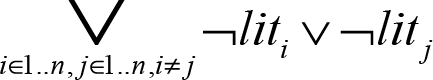We can also use the fact that both conjunction and disjunction are commutative (there is no difference between x ˄ y and y ˄ x ) to halve the number of clauses we create, as we only need to consider literal pairs where i < j .

Now that we know how to limit the number of ‘true’ literals to both at least 1 and at most 1, limiting the number of ‘true’ literals to exactly 1 is trivial; just apply both constraints at the same time via conjunction.

## C++ implementation

Now that we know how to describe Sudoku as a set of boolean clauses in CNF, we can implement a C++ code that uses this knowledge to solve arbitrary Sudoku. For brevity, this post will only contain relevant excerpts, but you can find the entire resulting code on GitHub 8 [ Sudoku ].

The first thing we need to solve is addressing variables, specifically converting a (row, column, value) triple into a specific value that represents it in the SAT solver. Because Sudoku is highly regular, we can get away with linearizing the three dimensions into one, and get the number of variable corresponding toas r × 9 × 9 + c × 9 + v . We can also use the fact that ``` Minisat::Var ``` is just a plain ``` int ``` numbered from 0 to avoid storing the variables at all because we can always compute the corresponding variable on-demand:

```  Minisat::Var toVar(int row, int column,
int value)
{
return row * columns * values
+ column * values + value;
}```

Now that we can quickly retrieve the SAT variable from a triplet of (row, column, value), but before we can use the variables, they need to be allocated inside the SAT solver:

```  void Solver::init_variables() {
for (int r = 0; r < rows; ++r) {
for (int c = 0; c < columns; ++c) {
for (int v = 0; v < values; ++v) {
static_cast<void>(solver.newVar());
}
}
}
}```

With the variables allocated, we can start converting the SAT version of Sudoku rules into C++ code.

• Rule 1 (No row contains duplicate numbers) is shown in Listing 3.

 ```for (int row = 0; row < rows; ++row) { for (int value = 0; value < values; ++value) { Minisat::vec literals; for (int column = 0; column < columns; ++column) { literals.push(Minisat::mkLit(toVar(row, column, value))); } exactly_one_true(literals); } } ``` Listing 3
• Rule 2 (No column contains duplicate numbers) is shown in Listing 4.

 ```for (int column = 0; column < columns; ++column) { for (int value = 0; value < values; ++value) { Minisat::vec literals; for (int row = 0; row < rows; ++row) { literals.push(Minisat::mkLit(toVar(row, column, value))); } exactly_one_true(literals); } } ``` Listing 4
• Rule 3 (None of the 3x3 boxes contain duplicate numbers)

This rule results in the most complex code, as it requires two iterations – one to iterate over all of the boxes and one to collect variables inside each box. However, the resulting code is still fairly trivial (see Listing 5).

 ```for (int r = 0; r < 9; r += 3) { for (int c = 0; c < 9; c += 3) { for (int value = 0; value < values; ++value) { Minisat::vec literals; for (int rr = 0; rr < 3; ++rr) { for (int cc = 0; cc < 3; ++cc) { literals.push(Minisat::mkLit(toVar (r + rr, c + cc, value))); } } exactly_one_true(literals); } } } ``` Listing 5
• Rule 4 (Each position contains exactly one number) is shown in Listing 6.

 ```for (int r = 0; r < 9; r += 3) { for (int c = 0; c < 9; c += 3) { for (int value = 0; value < values; ++value) { Minisat::vec literals; for (int rr = 0; rr < 3; ++rr) { for (int cc = 0; cc < 3; ++cc) { literals.push(Minisat::mkLit(toVar( r + rr, c + cc, value))); } } exactly_one_true(literals); } } } for (int row = 0; row < rows; ++row) { for (int column = 0; column < columns; ++column) { Minisat::vec literals; for (int value = 0; value < values; ++value) { literals.push(Minisat::mkLit(toVar( row, column, value))); } exactly_one_true(literals); } } ``` Listing 6

We also need to define the ``` exactly_one_true ``` helper (Listing 7).

 ```void Solver::exactly_one_true( Minisat::vec const& literals) { solver.addClause(literals); for (size_t i = 0; i < literals.size(); ++i) { for (size_t j = i + 1; j < literals.size(); ++j){ solver.addClause(~literals[i], ~literals[j]); } } } ``` Listing 7

With these snippets, we have defined a model of Sudoku as SAT. There are still 2 pieces of the solver missing: a method to specify values in the pre-filled positions of the board and a method that extracts the found solution to the puzzle.

Fixing the values in specific positions is easy, we can just add a unary clause for each specified position (see Listing 8).

 ```bool Solver::apply_board(board const& b) { for (int row = 0; row < rows; ++row) { for (int col = 0; col < columns; ++col) { auto value = b[row][col]; if (value != 0) { solver.addClause(Minisat::mkLit(toVar(row, col, value - 1))); } } } return ret; } ``` Listing 8

Because the only way to satisfy a unary clause is to set the appropriate variable to the polarity of the contained literal, this forces the specific position to always contain the desired value.

To retrieve a solution, we need to be able to determine a position’s value. Because only one of the variables for any given position can be set to true, the value corresponding to that specific variable is the value of the given position (see Listing 9).

 ```board Solver::get_solution() const { board b(rows, std::vector(columns)); for (int row = 0; row < rows; ++row) { for (int col = 0; col < columns; ++col) { for (int val = 0; val < values; ++val) { if (solver.modelValue(toVar(row, col, val)).isTrue()) { b[row][col] = val + 1; break; } } } } return b; } ``` Listing 9

With the solver finished, we can go on to benchmarking its performance.

## Benchmarks

As far as I could tell from a cursory search, there are no standard test suites for benchmarking Sudoku solvers. I decided to follow Peter Norvig’s blog post [ Norvig ] about his own Sudoku solver and use a set of 95 hard Sudokus [ Sudoku-data ]for measuring the performance of my solver.

The measurements were done on PC with factory-clocked i5-6600K CPU @ 3.5 GHz, the code was compiled using g++ under Windows Subsystem for Linux, and each input was run 10 times. After that, I took the mean of the results for each problem and put all of them into a boxplot. Since I am a proponent of LTO builds, I also compiled the whole thing, including MiniSat, with LTO enabled, and then benchmarked the binary.

Figure 1 shows the results.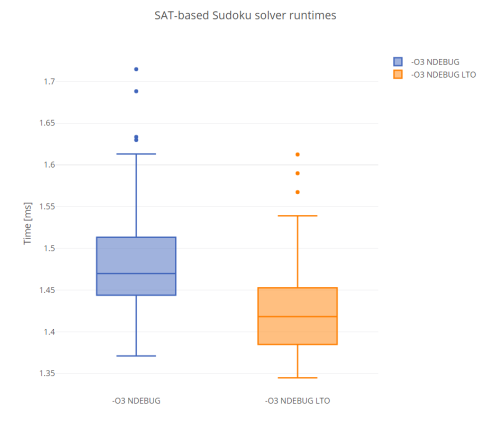Figure 1

As you can see, the LTO build performed somewhat better, but not significantly so. What is interesting, is that the number of outliers above the box, and the relative lengths of the whiskers, suggest that the underlying distribution of solver’s running time over all of the inputs is heavy-tailed. This means that the longest-running inputs will need significantly longer to be solved than the others, and it is a common attribute of solvers for NP-complete problems. This is because a single wrong decision during the search for a solution can lengthen the total runtime significantly.

There is one more question to answer, namely, how does this performance compare with high-performance Sudoku-specialized solvers? I picked two, ZSolver [ Zhouyundong12 ] and fsss2 [ Dobrichev ], and tried running them on the same set of problems. Not too surprisingly, they both outperformed our SAT-based solver badly. The sort of ‘converting’ solver we wrote will always be slower than a well-tuned specialised solver, but they do have some advantages that can make them desirable. As an example, I have no prior domain-specific knowledge about solving Sudokus, but I was able to write the SAT-based Sudoku solver in less than 2 hours. It is also much more readable and extendable 9 .

That is all for part 1, but I have much more I want to say about SAT solvers, so you can expect more posts on my blog both about using them, and about their internals and the theory behind why are they so fast.

This article was first published on Martin Hořeňovský’s blog, The Coding Nest , on 3 August 2018 at https://codingnest.com/modern-sat-solvers-fast-neat-underused-part-1-of-n/

## References

[Dobrichev] fsss2: https://github.com/dobrichev/fsss2

[Norvig] Peter Norvig ‘Solving Every Soduko Puzzle’, available at: http://norvig.com/sudoku.html

[Sudoku] Sudoku example code: https://github.com/horenmar/sudoku-example

[vcpkg] Installation of MiniSAT: https://github.com/Microsoft/vcpkg

[Wikipedia-1] ‘Conjunctive normal form’: https://en.wikipedia.org/wiki/Conjunctive_normal_form

[Wikipedia-2] ‘Sudoku’: https://en.wikipedia.org/wiki/Sudoku

[Zhouyundong12] ZSolver: http://forum.enjoysudoku.com/3-77us-solver-2-8g-cpu-testcase-17sodoku-t30470-90.html#p216748

1. This means polynomial, because when it comes to complexity theory, algorithms with polynomial complexity are generally considered tractable, no matter how high the exponent in the polynomial is, and algorithms with exponential complexity are considered intractable.
• To install a package, all its dependencies must be installed
• A package can list specific versions of other packages as dependencies
• Dependency sets of each version of a package can be different
• Only one version of a package can be installed
2. In fact, various dependency managers in the wild already use SAT solvers, such as Fedora’s DNF, Eclipse’s plugin manager, FreeBSD’s pkg, Debian’s apt (optionally), and others.
3. There are some extensions like XOR-SAT, which lets you natively encode XOR clauses, but these are relatively rare and only used in specialist domain, e.g. cryptanalysis.
4. When using the library interface of MiniSat, it defaults to being entirely deterministic. This means that if you are using the same version of MiniSat, the result will always be the same, even though there are different models.
5. Two formulas, f1 and f2, are equisatisfiable when f1 being satisfied means that f2 is also satisfied and vice versa.
6. There is also a notion of generalised sudoku, where you have to fill in numbers 1-N in NxN grid according to the same rules. It is proven to be NP-complete.
7. The real code differs in places, especially in that it is coded much more defensively and contains more validity checking in the form of assertions.
8. Try reading through the code of the linked solvers and imagine extending them to work with 18x18 boards. With our solver, it is just a matter of changing 3 constants on top of a file from 9 to 18.